# Financial Resources Formulary

Zur Navigation springen Zur Suche springen

by Clemens Werkmeister## Present values, perpetuities and annuities

Present value PV: value of a future payment Ct (in year t), discounted to year 0: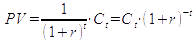Future value FVt: value of a present payment (in year 0), calculated by compounding to year t: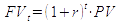t year
r discount rate (interest rate)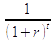discount factor with discount rate (interest rate) r for t years
Ct cash flow in year t
C0 initial investment of a project (for normal investment projects: C0 < 0)
T number of years of the project

The sum of several present values is a PV, too (additivity of present values):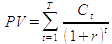Net present value NPV: PV of future payments (of a project or a company) plus the - usually negative - initial investment C0: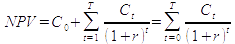Perpetuity (console): a periodic (annual) payment C that is received or paid forever (beginning with the first payment at the end of year 1):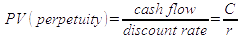Annuity: a payment of a level cash flow C during a specified number of years (from year 1 to n). Its present value can be calculated as difference between two perpetuities: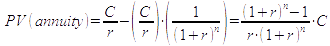Annuity (recovery) factor: average payment at the end of n periods, corresponding to a present value PV and considering for interest rate r: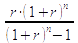Annuity present value factor: factor for the PV of n equal payments at the end of years 1 to n: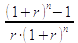The annuity C for years 1 to n corresponding to a present value PV and discount rate r is: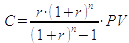Growing perpetuity: a perpetuity starting with cash flow C1 in year 1 and increasing by the annual growth rate g forever: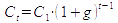(for t = 1, 2, …, ∞ ;g < r) and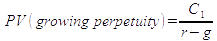Growing annuity: an annuity starting with cash flow C1 in year 1 and increasing by the annual growth rate g for n years: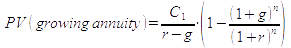(with t = 1, 2, …, n)

Internal rate of return (IRR): discount rate that results in NPV = 0: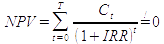Profitability index: ratio of NPV to investment of a project: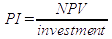Equivalent annual cash flow (EAC): cash flow per year with the same present value as the actual cash flow of the project: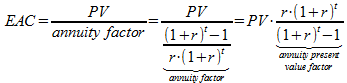## Interest and discount rates

Effective annual rate (EAR): annualized rate of shorter period interest rates (monthly, daily rates) using compound interest:

- EAR for a monthly rate m: EAR = (1+m)12 – 1
- EAR for a daily rate d: EAR = (1+d)360 – 1 (for 360 days per year)

Given an annual percentage rate (APR) of r, the corresponding EAR with respect to n shorter periods of equal length is: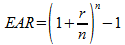Effective annual rate with continuous compounding: effective annual rate for n → ∞ shorter periods: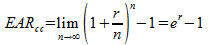(being r the simple annual rate)

Annual percentage rate (APR) or simple rate: annualized rate of shorter period interest rates (monthly, daily rates) using simple interest.

- APR for a monthly rate m: APR = 12 * m

Real rate of return: rate of return adjusted for inflation: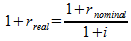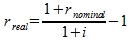(being i the inflation rate)

## Valuing bonds

Price of a bond: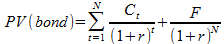with

Ct annual coupon interest payment
F face value (or principal)
r discount rate (yield to maturity)
N maturity

Duration of a bond with maturity N: weighted average period of bond payments: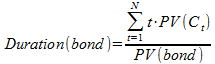Modified duration: a measure of volatility (elasticity) of bond prices: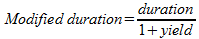## Valuing stocks

(Expected) Stock return r (equity cost of capital):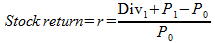Present value of growth opportunities (PVGO): net present value of a firm's future investments.

Return (Equity cost of capital) of a perpetual stream of dividends with growth: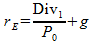Return on Equity with market values: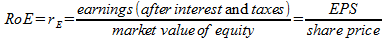Payout ratio: fraction of earnings paid out as dividends: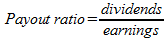Plowback ratio: fraction of earnings retained by the firm: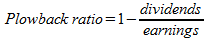Sustainable growth rate: rate at which a firm can steadily grow: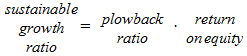Discounted cash flow (DCF): value of the free cash flows that are available to investors plus company value at the planning horizon, all discounted to present: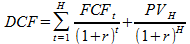## Risk and return

Risk premium of an asset: asset return – return of risk-free asset.

Variance: expected value of squared deviations of observations from their expected value (mean):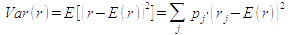(based on j observations)

Standard deviation: a measure of volatility of expected stock returns: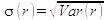Expected portfolio return (with two assets):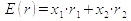Expected portfolio return (with j = 1, …, n assets):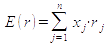xj weight of asset j in the portfolio
rj (expected) return of asset j

Variance of portfolio return (portfolio variance) in the case of two assets: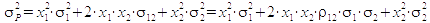Covariance between asset i and j with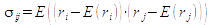Correlation coefficient between asset i and j: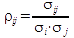Variance of portfolio return (portfolio variance) in the case of n assets: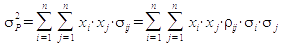Sharpe-Ratio: ratio of risk premium to risk (standard deviation):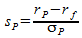Beta of the return of asset j to the market return (return of market portfolio m):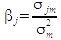Expected return following the security market line equation (SML):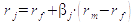Expected return of a stock in event studies: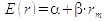Abnormal return = actual return – expected return =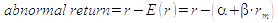## Capital Structure and Return

Weighted average cost of capital (WACC):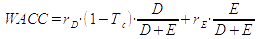rD interest rate on debt resp. debt cost of capital
rE return on equity resp. equity cost of capital
Tc corporate tax rate

Weighted average cost of capital (WACC) with a zero-tax rate: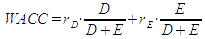Leverage-formula for return on equity: return on equity increases with debt/equity-ratio: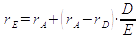Leverage-formula for equity beta: risk increases with debt/equity-ratio: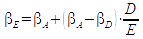## Exercises

Please try our Financial Exercises or have a look at the Financial Ratios or at our investment pages.